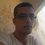# Who can handle this integral ?

$\int\limits_0^{\frac{\pi}{2}}\arctan\left(1-\cos^2 x\sin^2 x\right)\ \mathrm{d}x=\frac{\pi^2}{4}-\pi\arctan\sqrt{\frac{\sqrt{2}-1}{2}}.$ Can someone prove this ?Note by Haroun Meghaichi
6 years, 11 months ago

This discussion board is a place to discuss our Daily Challenges and the math and science related to those challenges. Explanations are more than just a solution — they should explain the steps and thinking strategies that you used to obtain the solution. Comments should further the discussion of math and science.

When posting on Brilliant:

• Use the emojis to react to an explanation, whether you're congratulating a job well done , or just really confused .
• Ask specific questions about the challenge or the steps in somebody's explanation. Well-posed questions can add a lot to the discussion, but posting "I don't understand!" doesn't help anyone.
• Try to contribute something new to the discussion, whether it is an extension, generalization or other idea related to the challenge.
• Stay on topic — we're all here to learn more about math and science, not to hear about your favorite get-rich-quick scheme or current world events.

MarkdownAppears as
*italics* or _italics_ italics
**bold** or __bold__ bold
- bulleted- list
• bulleted
• list
1. numbered2. list
1. numbered
2. list
Note: you must add a full line of space before and after lists for them to show up correctly
paragraph 1paragraph 2

paragraph 1

paragraph 2

[example link](https://brilliant.org)example link
> This is a quote
This is a quote
    # I indented these lines
# 4 spaces, and now they show
# up as a code block.

print "hello world"
# I indented these lines
# 4 spaces, and now they show
# up as a code block.

print "hello world"
MathAppears as
Remember to wrap math in $$ ... $$ or $ ... $ to ensure proper formatting.
2 \times 3 $2 \times 3$
2^{34} $2^{34}$
a_{i-1} $a_{i-1}$
\frac{2}{3} $\frac{2}{3}$
\sqrt{2} $\sqrt{2}$
\sum_{i=1}^3 $\sum_{i=1}^3$
\sin \theta $\sin \theta$
\boxed{123} $\boxed{123}$

## Comments

Sort by:

Top Newest

\displaystyle \begin{aligned} \int_0^{\pi/2} \arctan\left(1-\cos^2x\sin^2x\right)\,dx & =\int_0^{\pi/2} \left(\frac{\pi}{2}-\arctan\left(\frac{1}{1-\sin^2x\cos^2x}\right)\right)\,dx \\ &=\frac{\pi^2}{4}-\int_0^{\pi/2} \arctan(\sin^2x)\,dx-\int_0^{\pi/2} \arctan(\cos^2x)\,dx \\ &=\frac{\pi^2}{4}-2\int_0^{\pi/2}\arctan(\cos^2x)\,dx \\ \end{aligned}

Consider

$\displaystyle I(a)=\int_0^{\pi/2} \arctan(a\,\cos^2x)\,dx$

\displaystyle \begin{aligned} \Rightarrow I'(a)&=\int_0^{\pi/2} \frac{\cos^2x}{1+a^2\cos^4x}\,dx \\ &= \int_0^{\pi/2} \frac{\sec^2x}{\sec^4x+a^2}\,dx\\ &= \int_0^{\pi/2} \frac{\sec^2x}{(1+\tan^2x)^2+a}\,dx\\ &=\int_0^{\infty} \frac{dt}{t^4+2t^2+a^2+1}\,\,\,\,\,\,\,(\tan x=t) \\ \end{aligned}

With the substitution $t\mapsto \dfrac{\sqrt{a^2+1}}{t}$,

$\displaystyle I'(a)=\frac{1}{\sqrt{a^2+1}}\int_0^{\infty} \frac{t^2}{t^4+2t^2+a^2+1}\,dt$

\displaystyle \begin{aligned} \Rightarrow I'(a) &=\frac{1}{2\sqrt{a^2+1}}\int_0^{\infty} \frac{\sqrt{a^2+1}+t^2}{t^4+2t^2+a^2+1}\,dt \\ &=\frac{1}{2\sqrt{a^2+1}}\int_0^{\infty} \frac{1+\frac{\sqrt{1+a^2}}{t^2}}{\left(t-\frac{\sqrt{a^2+1}}{t}\right)^2+2(1+\sqrt{a^2+1})}\,dt\\ &=\frac{1}{2\sqrt{a^2+1}}\int_{-\infty}^{\infty} \frac{dy}{y^2+2(1+\sqrt{a^2+1})}\,\,\,\,\,\,\,\left(t-\frac{\sqrt{a^2+1}}{t}=y\right) \\ &=\frac{\pi}{2\sqrt{2}} \frac{1}{\sqrt{1+a^2}\sqrt{1+\sqrt{a^2+1}}} \\ \end{aligned}

Integrating back,

\displaystyle \begin{aligned} I(1)-I(0)=I(1) &=\frac{\pi}{2\sqrt{2}}\int_0^1 \frac{da}{\sqrt{1+a^2}\sqrt{1+\sqrt{a^2+1}}} \\ &= \frac{\pi}{2\sqrt{2}}\int_1^{\sqrt{2}} \frac{dt}{\sqrt{t-1}(t+1)}\,\,\,\,\,\,\,(\sqrt{a^2+1}=t) \\ &=\frac{\pi}{\sqrt{2}}\int_0^{\sqrt{\sqrt{2}-1}} \frac{du}{u^2+2} \,\,\,\,\,\,\,(t-1=u^2) \\ &=\frac{\pi}{2} \left(\arctan\frac{u}{\sqrt{2}}\right|_0^{\sqrt{\sqrt{2}-1}} \\ &=\frac{\pi}{2}\arctan\left(\sqrt{\frac{\sqrt{2}-1}{2}}\right) \\ \end{aligned}

Hence,

$\boxed\int_0^{\pi/2} \arctan\left(1-\cos^2x\sin^2x\right)\,dx=\dfrac{\pi^2}{4}-\pi\arctan\left(\sqrt{\dfrac{\sqrt{2}-1}{2}}\right)}$

- 6 years, 11 months ago

Log in to reply

What a great job! Hats off to you @Pranav Arora .

- 6 years, 11 months ago

Log in to reply

Wonderful solution Pranav!!! How do you think of such things?!$\ddot\smile$

- 6 years, 11 months ago

Log in to reply

Thank you Karthik! :)

I don't think I did anything special in this one, I used only the standard techniques as you can see. I am sure you too would have solved it if you jumped on this one before me. ;)

- 6 years, 11 months ago

Log in to reply

Oh, no...I tried a lot but did not succeed. My approach was also 'differentiating under the integral' but I was nowhere close to the solution. Great solution once again!$\ddot\smile$

- 6 years, 11 months ago

Log in to reply

Excellent!!!

- 6 years, 10 months ago

Log in to reply

What level calculus is this..?I am in 12th grade am I supposed to be able to solve this...??

- 6 years, 9 months ago

Log in to reply

I suppose this is problem solving calculus. You don't regularly learn stuff this hard in school.

- 6 years, 9 months ago

Log in to reply

Hey are you actually 14 year old...??

- 6 years, 9 months ago

Log in to reply

×

Problem Loading...

Note Loading...

Set Loading...